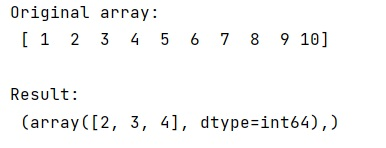# How to use numpy.where() with logical operators?

Learn, how to use numpy.where() with logical operators in Python?
Submitted by Pranit Sharma, on February 16, 2023

NumPy is an abbreviated form of Numerical Python. It is used for different types of scientific operations in python. Numpy is a vast library in python which is used for almost every kind of scientific or mathematical operation. It is itself an array which is a collection of various methods and functions for processing the arrays.

## Using numpy.where() with logical operators

The numpy.where() returns a tuple of length equal to the dimension of the numpy ndarray on which it is called (in other words ndim) and each item of tuple is a numpy ndarray of indices of all those values in the initial ndarray for which the condition is True.

Suppose that we are given with a numpy array and we need to find the indices of all elements in an array that are greater than a specific value a and less than a specific value b.

For this purpose, we will simply use numpy.where() clause and pass two conditions and also apply the logical and operator in between. Note that we will use & operator rather than the keyword and.

Let us understand with the help of an example,

## Python code to demonstrate the use numpy.where() with logical operators

```# Import numpy
import numpy as np

# Creating a numpy array
arr = np.array([1,2,3,4,5,6,7,8,9,10])

# Display original array
print("Original array:\n",arr,"\n")

# selecting array values using
# where and logical operator
res = np.where((arr > 2) & (arr < 6))

# Display Result
print("Result:\n",res)
```

Output: